• Introduction
• What is in this manual
• What is Caspoc
• User interface
• Introduction
• Starting
• Simulation
• Editing
• Viewing and printing
• Getting Started
• Basic editing
• Simulation in the time domain
• Basic User Interface Topics
• Editing
• Simulation
• Viewing
• Library
• Reports
• Project management
• Circuit and Block Diagram Components
• Introduction
• Cscript and user defined functions
• Component parameters
• Modeling Topics
• Introduction
• Power Electronics
• Semiconductors
• Electrical Machines
• Electrical drives
• Power Systems
• Mechanical Systems
• Thermal Systems
• Magnetic Circuits
• Green Energy
• Coupling to FEM
• Experimenter
• Analog hardware description language
• Embedded C code Export
• Coupling to Spice
• Small Signal Analysis
• Matlab coupling
• Tips and tricks
• Appendices

## Transmission lines.

There are essentially two different types of models for transmission lines. One is based on segmented circuit models and the second is based on a transmission line. The models based on the transmission line are more accurate, but require more simulation time. The segmented lines are given as a π section line (DistributedLine) and T segment (DistributedCable). The T segment line is only given as a three phase model.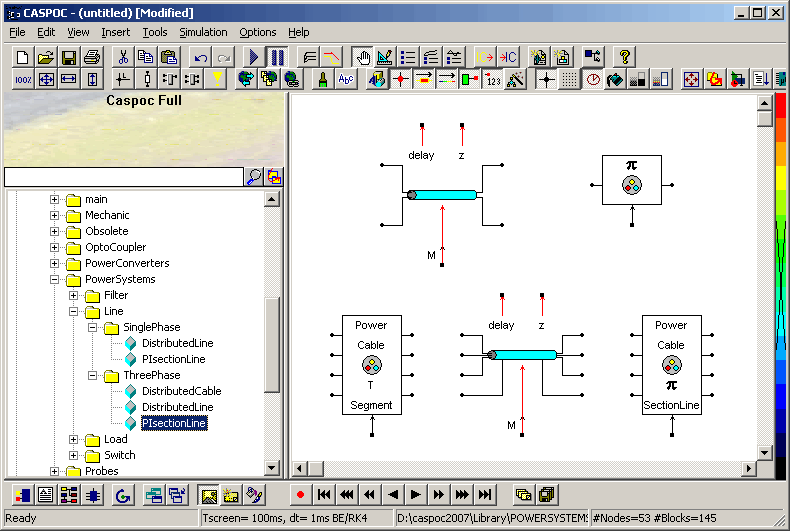### PI Section Line

The PI Section Line block implements a single-phase transmission line with parameters lumped in PI sections.

For a transmission line, the resistance, inductance, and capacitance are uniformly distributed along the line. An approximate model of the distributed parameter line is obtained by cascading several identical PI sections, as shown in the following figure.Unlike the Distributed Parameter Line block, which has an infinite number of states, the PI section linear model has a finite number of states that permit you to compute a linear state-space model. The number of sections to be used depends on the frequency range to be represented.

A good approximation of the maximum frequency range represented by the PI line model is given by the following equation:

fmax=Nv/8l where
 N Number of PI sections v Propagation speed in km/s = 1/SQRT(LC) L in H/km, C in F/km l Line length in km

For example, for a 100 km aerial line having a propagation speed of 300,000 km/s, the maximum frequency range represented with a single PI section is approximately 375 Hz. For studying interactions between a power system and a control system, this simple model could be sufficient. However for switching surge studies involving high-frequency transients in the kHz range, much shorter PI sections should be used. In fact, you can obtain the most accurate results by using a distributed parameters line model.

### Parameters PI Section Line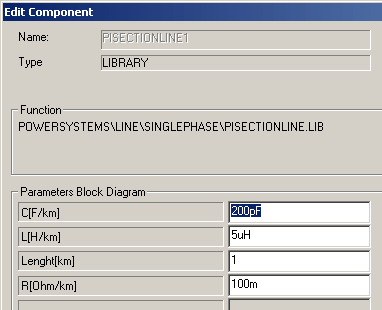Resistance per unit length The resistance R per unit length, in ohms/km (Ω/km). Inductance per unit length The inductance per unit length, in henries/km (H/km). Capacitance per unit length The capacitance C per unit length, in farads/km(F/km). Line length The line length, in km.

### Distributed Cable T segment

The distributed cable T segment differs from the pi section line in the way the circuit components are organizedDepending on the number of sections that you place in series, you have to provide the parameter n in this model, specifying the number of sectionsin series. So summarizing, if you place three DistributedCable blocks in series, you have to set n equal to 3.

### Parameters Distributed Cable T Segment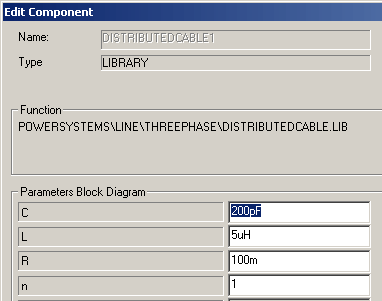Resistance The resistance R for the total line, in ohms (Ω). Inductance The inductance for the total line, in henries (H). Capacitance The capacitance C for the total line, in farads (F). Number of segments The numberof segments that are placed in series.

### Distributed Parameter Line with lumped losses

The Distributed Parameter Line block implements a distributed parameter line model with lumped losses. The model is based on the Bergeron's traveling wave method. In this model, the lossless distributed LC line is characterized by two values (for a single-phase line): the surge impedance ZC=SQRT(L/C) and the phase velocity v=1/SQRT(LC).

The model uses the fact that the quantity e+Zi (where e is line voltage and i is line current) entering one end of the line must arrive unchanged at the other end after a transport delay of τ=d/v, where d is the line length. By lumping R/4 at both ends of the line and R/2 in the middle, the following two-port model is derived.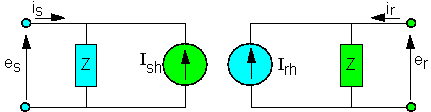where

Z=ZC+R/4 ZC=SQRT(L/C) h=(ZC-R/4)/(ZC+R/4) τ=d*SQRT(LC)

In comparison to the PI section line model, the distributed line represents wave propagation phenomena and line end reflections with much better accuracy.

### Parameters Distributed Parameter Line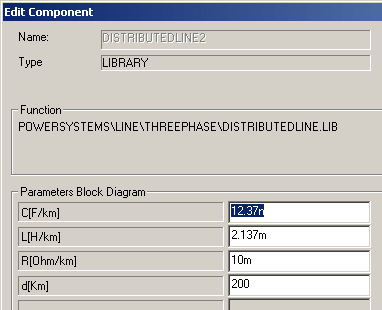Resistance per unit length The resistance R per unit length, in ohms/km (Ω/km). Inductance per unit length The inductance per unit length, in henries/km (H/km). Capacitance per unit length The capacitance C per unit length, in farads/km(F/km). Line length The line length, in km.